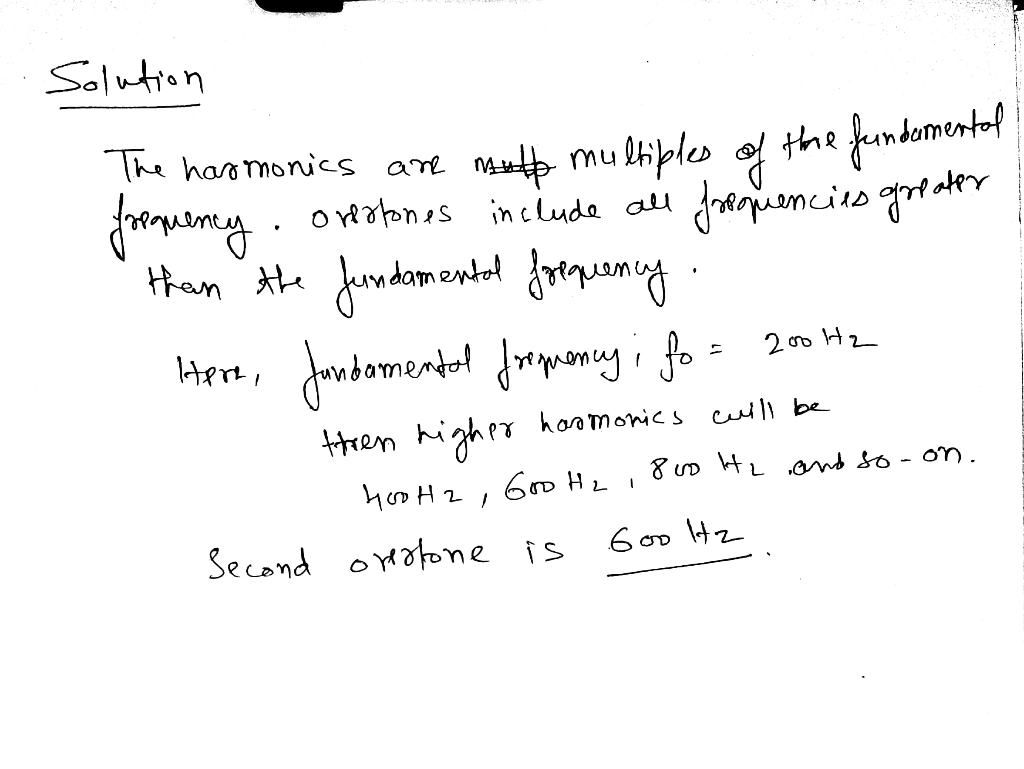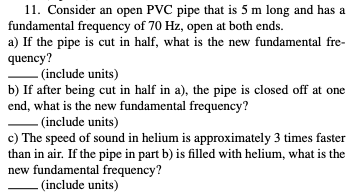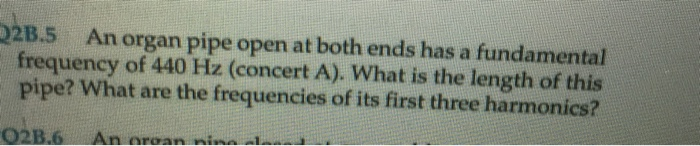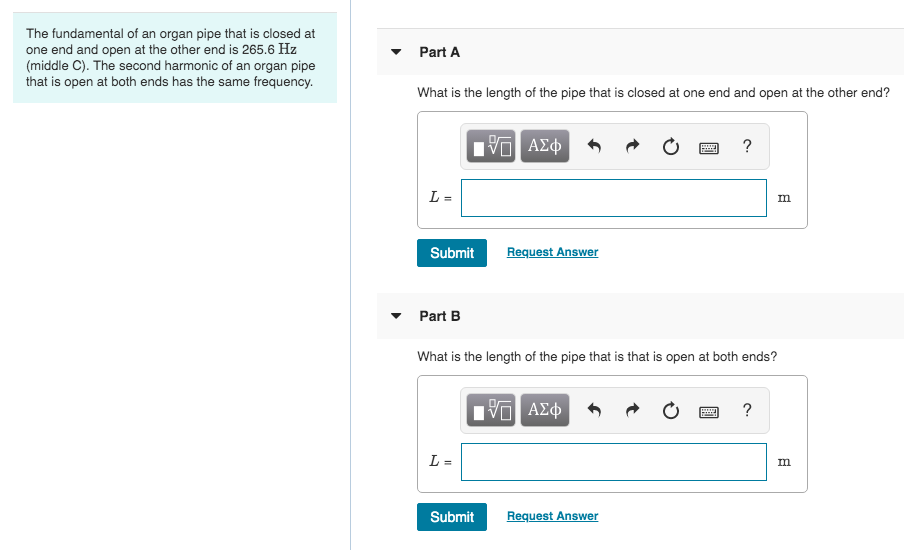Question

# If an open-open pipe resonator has a fundamental frequency of 200Hz , what is the frequency...

If an open-open pipe resonator has a fundamental frequency of 200Hz , what is the frequency of its second overtone?#### Earn Coins

Coins can be redeemed for fabulous gifts.

Similar Homework Help Questions
• ### If an open-open pipe resonator has a fundamental frequency of 200Hz, what is the frequency of...

If an open-open pipe resonator has a fundamental frequency of 200Hz, what is the frequency of its second overtone?

• ### What is the frequency of the second overtone of a closed-pipe resonator with a length of...

What is the frequency of the second overtone of a closed-pipe resonator with a length of 22.0cm? (Assume the speed of sound is 331m/s.)

• ### (a) What length of pipe open at both ends has a fundamental frequency of 3.75 102...

(a) What length of pipe open at both ends has a fundamental frequency of 3.75 102 Hz? Find the first overtone. lpipe = .457 Incorrect: m fovertone = 750 Correct: Hz (b) If the one end of this pipe is now closed, what is the new fundamental frequency? Find the first overtone. ffundamental = 750 Incorrect: Hz fovertone = 615 Incorrect: Hz (c) If the pipe is open at one end only, how many harmonics are possible in the normal...

• ### Organ pipe A with both ends open has a fundamental frequency of 320.0 Hz. The third...

Organ pipe A with both ends open has a fundamental frequency of 320.0 Hz. The third harmonic of organ pipe B with one end open has the same frequency as the second harmonic of pipe A. Assume a speed of sound of 343 m/s. What is the length of Pipe A? What is the length of Pipe B?

• ### A pipe open only at one end has a fundamental frequency of 254 Hz. A second...

A pipe open only at one end has a fundamental frequency of 254 Hz. A second pipe, initially identical to the first pipe, is shortened by cutting off a portion of the open end. Now when both pipes vibrate at their fundamental frequencies, a beat frequency of 20 Hz is heard. How many centimeters were cut off the end of the second pipe? The speed of sound is 345 m/s.

• ### A pipe open only at one end has a fundamental frequency of 240 Hz. A second...

A pipe open only at one end has a fundamental frequency of 240 Hz. A second pipe, initially identical to the first pipe, is shortened by cutting off a portion of the open end. Now when both pipes vibrate at their fundamental frequencies, a beat frequency of 16 Hz is heard. How many centimeters were cut off the end of the second pipe? The speed of sound is 345 m/s.

• ### 11. Consider an open PVC pipe that is 5 m long and has a fundamental frequency...11. Consider an open PVC pipe that is 5 m long and has a fundamental frequency of 70 Hz, open at both ends. a) If the pipe is cut in half, what is the new fundamental fre- quency? (include units) b) If after being cut in half in a), the pipe is closed off at one end, what is the new fundamental frequency? (include units) c) The speed of sound in helium is approximately 3 times faster than in air....

• ### On a day when the speed of sound in air is 345 m/s, the fundamental frequency...

On a day when the speed of sound in air is 345 m/s, the fundamental frequency of an open-ended pipe is 690 Hz. If the second harmonic of this pipe has the same wavelength as the second overtone (third harmonic) of a closed-end pipe, what is the length of each pipe?

• ### 2B.5 An organ pipe open at both ends has a fundamental frequency of 440 Hz (concert...2B.5 An organ pipe open at both ends has a fundamental frequency of 440 Hz (concert A). What is the length of this pipe? What are the frequencies of its first three harmonics? 02B.6 An aroan nina

• ### The fundamental of an organ pipe that is closed at one end and open at the...The fundamental of an organ pipe that is closed at one end and open at the other end is 265.6 Hz (middle C). The second harmonic of an organ pipe that is open at both ends has the same frequency. Part A What is the length of the pipe that is closed at one end and open at the other end? PO AJĄ O O ? Submit Request Answer Part B What is the length of the pipe that is...